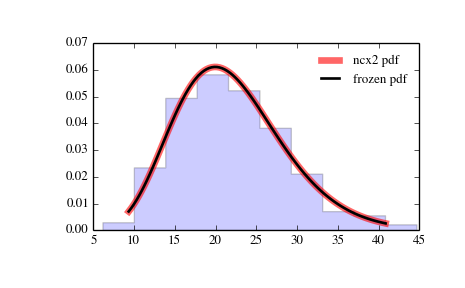# scipy.stats.ncx2¶

scipy.stats.ncx2 = <scipy.stats._continuous_distns.ncx2_gen object at 0x2b45d3000f50>[source]

A non-central chi-squared continuous random variable.

Continuous random variables are defined from a standard form and may require some shape parameters to complete its specification. Any optional keyword parameters can be passed to the methods of the RV object as given below:

Parameters: x : array_like quantiles q : array_like lower or upper tail probability df, nc : array_like shape parameters loc : array_like, optional location parameter (default=0) scale : array_like, optional scale parameter (default=1) size : int or tuple of ints, optional shape of random variates (default computed from input arguments ) moments : str, optional composed of letters [‘mvsk’] specifying which moments to compute where ‘m’ = mean, ‘v’ = variance, ‘s’ = (Fisher’s) skew and ‘k’ = (Fisher’s) kurtosis. (default=’mv’) Alternatively, the object may be called (as a function) to fix the shape, location, and scale parameters returning a “frozen” continuous RV object: rv = ncx2(df, nc, loc=0, scale=1) Frozen RV object with the same methods but holding the given shape, location, and scale fixed.

Notes

The probability density function for ncx2 is:

```ncx2.pdf(x, df, nc) = exp(-(nc+df)/2) * 1/2 * (x/nc)**((df-2)/4)
* I[(df-2)/2](sqrt(nc*x))
```

for x > 0.

Examples

```>>> from scipy.stats import ncx2
>>> import matplotlib.pyplot as plt
>>> fig, ax = plt.subplots(1, 1)
```

Calculate a few first moments:

```>>> df, nc = 21, 1.05604659751
>>> mean, var, skew, kurt = ncx2.stats(df, nc, moments='mvsk')
```

Display the probability density function (pdf):

```>>> x = np.linspace(ncx2.ppf(0.01, df, nc),
...               ncx2.ppf(0.99, df, nc), 100)
>>> ax.plot(x, ncx2.pdf(x, df, nc),
...          'r-', lw=5, alpha=0.6, label='ncx2 pdf')
```

Alternatively, freeze the distribution and display the frozen pdf:

```>>> rv = ncx2(df, nc)
>>> ax.plot(x, rv.pdf(x), 'k-', lw=2, label='frozen pdf')
```

Check accuracy of cdf and ppf:

```>>> vals = ncx2.ppf([0.001, 0.5, 0.999], df, nc)
>>> np.allclose([0.001, 0.5, 0.999], ncx2.cdf(vals, df, nc))
True
```

Generate random numbers:

```>>> r = ncx2.rvs(df, nc, size=1000)
```

And compare the histogram:

```>>> ax.hist(r, normed=True, histtype='stepfilled', alpha=0.2)
>>> ax.legend(loc='best', frameon=False)
>>> plt.show()
```Methods

 rvs(df, nc, loc=0, scale=1, size=1) Random variates. pdf(x, df, nc, loc=0, scale=1) Probability density function. logpdf(x, df, nc, loc=0, scale=1) Log of the probability density function. cdf(x, df, nc, loc=0, scale=1) Cumulative density function. logcdf(x, df, nc, loc=0, scale=1) Log of the cumulative density function. sf(x, df, nc, loc=0, scale=1) Survival function (1-cdf — sometimes more accurate). logsf(x, df, nc, loc=0, scale=1) Log of the survival function. ppf(q, df, nc, loc=0, scale=1) Percent point function (inverse of cdf — percentiles). isf(q, df, nc, loc=0, scale=1) Inverse survival function (inverse of sf). moment(n, df, nc, loc=0, scale=1) Non-central moment of order n stats(df, nc, loc=0, scale=1, moments=’mv’) Mean(‘m’), variance(‘v’), skew(‘s’), and/or kurtosis(‘k’). entropy(df, nc, loc=0, scale=1) (Differential) entropy of the RV. fit(data, df, nc, loc=0, scale=1) Parameter estimates for generic data. expect(func, df, nc, loc=0, scale=1, lb=None, ub=None, conditional=False, **kwds) Expected value of a function (of one argument) with respect to the distribution. median(df, nc, loc=0, scale=1) Median of the distribution. mean(df, nc, loc=0, scale=1) Mean of the distribution. var(df, nc, loc=0, scale=1) Variance of the distribution. std(df, nc, loc=0, scale=1) Standard deviation of the distribution. interval(alpha, df, nc, loc=0, scale=1) Endpoints of the range that contains alpha percent of the distribution

#### Previous topic

scipy.stats.nakagami

scipy.stats.ncf